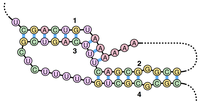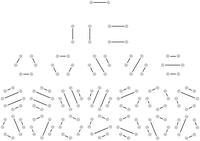# Catalan Numbers and RNA Secondary Structures solved by 1410

March 16, 2013, 6:22 a.m. by Rosalind Team

## The Human KnotFigure 2. This pseudoknot was formed when bonding occurred at the endpoints of overlapping intervals [1,3] and [2, 4]. (Courtesy: Sakurambo, Wikimedia Commons User)

You may have had the misfortune to participate in a team-building event that featured the "human knot," in which everyone joins hands with two other people, and the group must undo the giant knot of arms without letting go (see Figure 1).

Let's consider a simplified version of the human knot. Say that we have an even number of people at a party who are standing in a circle, and they pair off and shake hands at the same time. One combinatorial question at hand asks us to count the total number of ways that the guests can shake hands without any two pairs interfering with each other by crossing arms.

This silly little counting problem is actually an excellent analogy for RNA folding. In practice, base pairing can occur anywhere along the RNA molecule, but the secondary structure of RNA often forbids base pairs crossing over each other, which forms a structure called a pseudoknot (see Figure 2)). Pseudoknots are not technically knots, but they nevertheless cause RNA to fold over itself.

Forbidding pseudoknots offers an interesting wrinkle to the problem of counting potential RNA secondary structures that we started working with in “Perfect Matchings and RNA Secondary Structures”, in which every possible nucleotide of a strand of RNA must base pair with another nucleotide.

## ProblemFigure 3. The only two noncrossing perfect matchings of basepair edges (shown in red) for the RNA string UAGCGUGAUCAC.Figure 4. All possible noncrossing perfect matchings in complete graphs on 2, 4, 6, and 8 nodes; the total number of such matchings are 1, 2, 5, and 14, respectively.

A matching in a graph is noncrossing if none of its edges cross each other. If we assume that the $n$ nodes of this graph are arranged around a circle, and if we label these nodes with positive integers between 1 and $n$, then a matching is noncrossing as long as there are not edges $\{i, j\}$ and $\{k, l\}$ such that $i < k < j < l$.

A noncrossing matching of basepair edges in the bonding graph corresponding to an RNA string will correspond to a possible secondary structure of the underlying RNA strand that lacks pseudoknots, as shown in Figure 3.

In this problem, we will consider counting noncrossing perfect matchings of basepair edges. As a motivating example of how to count noncrossing perfect matchings, let $c_n$ denote the number of noncrossing perfect matchings in the complete graph $K_{2n}$. After setting $c_0 = 1$, we can see that $c_1$ should equal 1 as well. As for the case of a general $n$, say that the nodes of $K_{2n}$ are labeled with the positive integers from 1 to $2n$. We can join node 1 to any of the remaining $2n - 1$ nodes; yet once we have chosen this node (say $m$), we cannot add another edge to the matching that crosses the edge $\{1, m\}$. As a result, we must match all the edges on one side of $\{1, m\}$ to each other. This requirement forces $m$ to be even, so that we can write $m = 2k$ for some positive integer $k$.

There are $2k - 2$ nodes on one side of $\{1, m\}$ and $2n - 2k$ nodes on the other side of $\{1, m\}$, so that in turn there will be $c_{k-1} \cdot c_{n - k}$ different ways of forming a perfect matching on the remaining nodes of $K_{2n}$. If we let $m$ vary over all possible $n - 1$ choices of even numbers between 1 and $2n$, then we obtain the recurrence relation $c_n = \sum_{k = 1}^{n}{c_{k-1} \cdot c_{n-k}}$. The resulting numbers $c_n$ counting noncrossing perfect matchings in $K_{2n}$ are called the Catalan numbers, and they appear in a huge number of other settings. See Figure 4 for an illustration counting the first four Catalan numbers.

Given: An RNA string $s$ having the same number of occurrences of 'A' as 'U' and the same number of occurrences of 'C' as 'G'. The length of the string is at most 300 bp.

Return: The total number of noncrossing perfect matchings of basepair edges in the bonding graph of $s$, modulo 1,000,000.

## Sample Dataset

>Rosalind_57
AUAU


## Sample Output

2


## Hint

Write a function that counts Catalan numbers via dynamic programming. How can we modify this function to apply to our given problem?